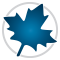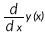Math Equation Editor - Maple Features - Maplesoft# Math Equation Editor

## Express complicated mathematical problems easily using standard mathematical notation

### With Maple's equation editor, you can express complicated mathematical problems easily using standard mathematical notation.

Intuitive, rapid problem entry

• Over 40 fill-in-the-blank templates are available for common operations, including integrals, derivatives, and function definitions.
• Automatic formatting for common commands: the ^ key automatically moves the cursor to the exponent position, and the / key creates a division bar and moves the cursor to the denominator.
• Symbol completion allows you to quickly insert a symbol.  You do not need to know the exact name; you only need to enter the first few characters of the symbol name.
• Palettes are conveniently docked beside your main workspace. You can expand, collapse, remove, and reorder palettes, so frequently used ones are easily accessible.
• Implicit multiplication: 3x  is the same as 3.x.

Math that “looks right”

• Supports multiple notations for the same concept. For example,,, y'(x), and y’ all represent the derivative of y with respect to x.
• Extensive layout control: superscripts, subscripts, over, under, pre-superscripts, and pre-subscripts.
• Over 1000 symbols are available.
• Typesetting rules can be modified to change the display and interpretation of particular commands and symbols.Maple’s math equation editor allows you to express complicated mathematical problems easily using standard mathematical notation.

### Equation Editor Demonstration

Maple's Equation Editor is an important component of Clickable Math, Maple's set of easy-to-use clickable interface features that allow you to focus on the concepts, not the tool.

Prêt(e) pour l'étape suivante ?

*Ce programme d'évaluation n'est proposé actuellement ni aux étudiants ni aux particuliers.

Achetez: